## Water turbines - notes on calculations

Figurerepresents a physical setting of a turbine casing with respect to a dam. Let us denote by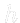the head, i.e. the difference between heights of water levels in front of the dam and at the back of it. Vertical distance between the center of mass of the inlet surface of volute and the high water level is denoted by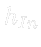. Vertical distance between the center of mass of the outlet surface of draft tube and the low water level is denoted by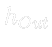. Finally, vertical distance between the centres of mass of inlet and outlet surfaces is denoted by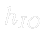.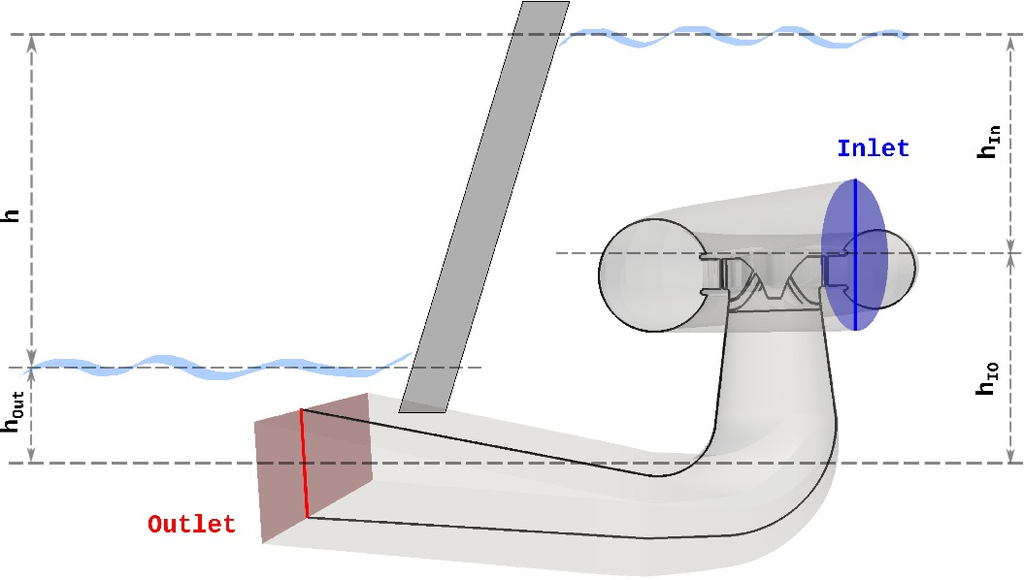Figure: General water turbine sketch. Physical setting of a turbine casing with respect to a dam. Water levels indicated.

We can see there are several simple relations among quantities just defined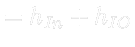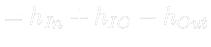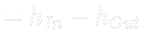To simplify calculations we usually considers water an incompressible fluid with no phase changes possible and we solve the well known equations, the conservation laws the fluid obeys.

Coservation of linear momentum is expressed by the Navier-Stokes equation. In this case (incompressible fluid, steady state) it can take the form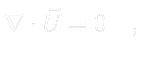where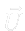is a velocity field,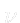kinematic viscosity,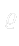density,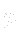pressure field and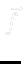is a force density of a gravitational field, given by (). Conservation of mass is expressed by the continuity equation. In this case it takes the simple formIt remains to discuss boundary conditions for the equations mentioned above. For we usually do not know the inlet velocity field, we are about to prescribe pressure field for both inlet and outlet surface. At the inlet surface a total pressure is known and it is equal to hydrostatic one. With the use of formula () we can write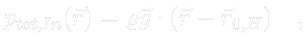where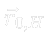is a position vector of an arbitrary point of the high water level (plane). Since total pressure is defined as a sum of static and dynamical pressure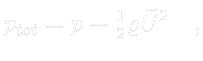we can, by means of this definition () and formula (), express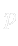at the inlet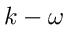At the outlet the situation is a little bit complicated. After the flow (with high total pressure) exits the draft tube it mixes with water (with low total pressure) surrounding the draft tube. We usually take simplifying steps and assume the static pressure of exiting flow equals the hydrostatic pressure of surrounding water, i.e. we write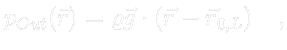where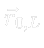is a position vector of an arbitrary point of the low water level. Described steps correspond to real physical setting. In a task like this we need to know not only the, but even(optionally), i.e. a position of the turbine with respect to the high or low water level. Quantitycan be obtained from the given turbine casing geometry, of course.